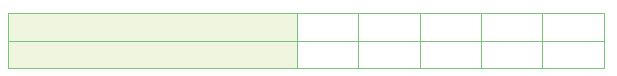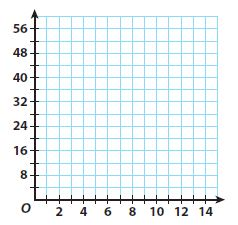Homework Explained - Math Practice 101Dear guest, you are not a registered member. As a guest, you only have read-only access to our books, tests and other practice materials.

As a registered member you can:

Registration is free and doesn't require any type of payment information. Click here to Register.
Go to page:
Chapter 4: Nonproportional Relationships; Lesson 1:Representing Linear Nonproportional Relationships

Independent Practice

State whether the graph of each linear relationship is a solid line or a set of unconnected points. Explain your reasoning.

• Question 7

The relationship between the number of $4 lunches you buy with a$100 school lunch card and the money remaining on the card

• Question 8

The relationship between time and the distance remaining on a 3-mile walk for someone walking at a steady rate of 2 miles per hour.

• Question 9

Analyze Relationships

Simone paid $12 for an initial year’s subscription to a magazine. The renewal rate is$8 per year. This situation can be represented by the equation y = 8x + 12, where x represents the number of years the subscription is renewed and y represents the total cost.

a. Make a table of values for this situation.• Type below:
• Question 9

b. Draw a graph to represent the situation. Include a title and axis labels.

• Type below:
• Question 9

c. Explain why this relationship is not proportional.• Type below:
• Question 9

d. Does it make sense to connect the points on the graph with a solid line? Explain.

• Type below:

Yes, email page to my online tutor. (if you didn't add a tutor yet, you can add one here)